# VBA Comparison Operators – Not Equal to & More

VBA allows you to use comparison operators to compare values. By using these operators, you can compare values and return a Boolean True or False as a result.

## The List of the Main Comparison Operators in VBA

First, we will display the list of comparison operators that can be used in VBA code:

 Comparison Operator Explanation = Equal to <> Not Equal to > Greater than >= Greater than or Equal to < Less than <= Less than or Equal to

## Equal To

The Equal to operator checks if two values are equal and returns True or False. Here is the example code:

In this example, we want to check if intA is equal to intB. If this is true, the value of Boolean blnResult will be True, otherwise, it will be False.

First, we set values of intA and intB to 5:

After that, we use the = operator in the If statement to check if the values of intA and intB are equal:

Both variables are equal to 5, therefore the blnResult returns True: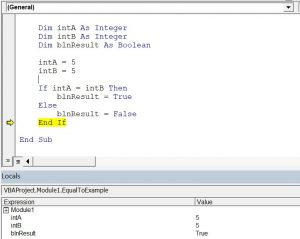Image 1. Using the Equal To operator

## Not Equal To

The Not Equal to operator checks if two values are not equal and returns True or False. Here is the example code:

In this example, we want to check if intA is not equal to intB. If this is true, the value of Boolean blnResult will be True, otherwise, it will be False.

We use the <> operator in the If statement to check if the values of intA and intB are different:

The value of intA is 5 and the value of intB is 6, the variables are not equal, therefore the blnResult returns True: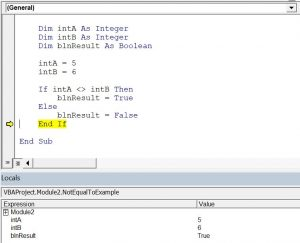Image 2. Using the Not Equal To operator

## Greater Than

The Greater than operator checks if the first value is greater than the second value and returns True or False. Here is the example code:

In this example, we want to check if intA is greater than intB. If this is true, the value of Boolean blnResult will be True, otherwise, it will be False.

Furthermore, we use the > operator in the If statement in order to check if the value of intA is greater than intB:

In conclusion, as intA is greater than intB, the blnResult returns True: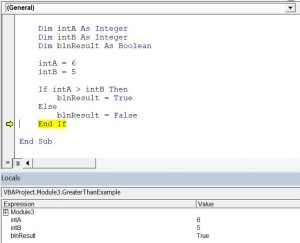Image 3. Using the Greater Than operator

## Greater Than or Equal To

The Greater than or Equal to operator checks if the first value is greater than or equal to the second value and returns True or False. Here is the example code:

In this example, we want to check if intA is greater than or equal to intB. If this is true, the value of Boolean blnResult will be True, otherwise, it will be False.

We use the >= operator in the If statement to check if the value of intA is greater than or equal to intB:

In conclusion, as both variables are equal to 5, the blnResult returns True: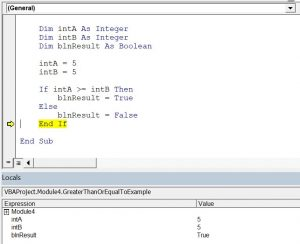Image 4. Using the Greater Than or Equal To operator

## Less Than

The Less than operator checks if the first value is less than the second value and returns True or False. Here is the example code:

In this example, we want to check if intA is less than intB. If this is true, the value of Boolean blnResult will be True, otherwise, it will be False.

We use the < operator in the If statement to check if the value of intA is less than intB:

As intA is less than intB, the blnResult returns True: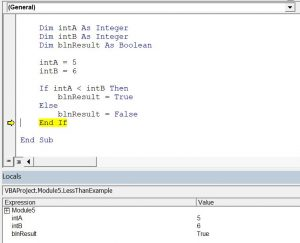Image 5. Using the Less Than operator

## Less Than or Equal To

The Less than or Equal to operator checks if the first value is less than or equal to and returns True or False. Here is the example code:

In this example, we want to check if intA is less than or equal to intB. If this is true, the value of Boolean blnResult will be True, otherwise, it will be False.

We use the <= operator in the If statement to check if the value of intA is less than or equal to intB:

In conclusion, as both variables are equal to 5, the blnResult returns True: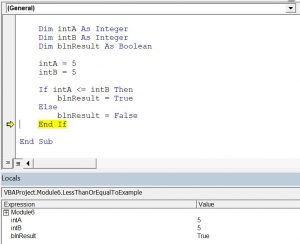Image 6. Using the Less Than or Equal To operator

## Is Operator

The Is Operator tests if two object variables contain the same object:

## Like Operator

The Like Operator can be used to find inexact text matches. This example will test if a string starts with “Mr.”

If you want to learn how to compare strings, click here: VBA Compare Strings – StrComp

If you want to learn how to use comparison operators, click here: VBA Logical Operators